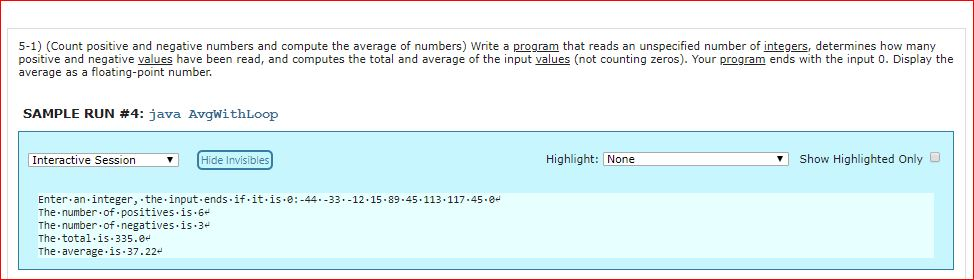# 5-1) (Count positive and negative numbers and compute the average of numbers) Write a program that reads an unspecified number of integers, determines how many positive and negative values have been read, and computes the total and average of the input values (not counting zeros). Your program ends with the input 0. Display the average as a floating-point number SAMPLE RUN #4: java AvgwithLoop Interactive SessionHide Invisibles Highlight: None Show Highlighted Only D Enter.an.integer, the.input ends.if.it.is.e:-4433.-12.15.89.45 The-number-of. positives is . 6 The number.of.negatives.is.3 The total.is.335.0 The average.is 37.22 113.117.45.8

Question

Use Java to answer the question.

5-1) (Count positive and negative numbers and compute the average of numbers)

Write a program that reads an unspecified number of integers, determines how many positive and negative values have been read, and computes the total and average of the input values (not counting zeros). Your program ends with the input 0. Display the average as a floating-point number.help_outlineImage Transcriptionclose5-1) (Count positive and negative numbers and compute the average of numbers) Write a program that reads an unspecified number of integers, determines how many positive and negative values have been read, and computes the total and average of the input values (not counting zeros). Your program ends with the input 0. Display the average as a floating-point number SAMPLE RUN #4: java AvgwithLoop Interactive SessionHide Invisibles Highlight: None Show Highlighted Only D Enter.an.integer, the.input ends.if.it.is.e:-4433.-12.15.89.45 The-number-of. positives is . 6 The number.of.negatives.is.3 The total.is.335.0 The average.is 37.22 113.117.45.8 fullscreen

### Want to see this answer and more?

Experts are waiting 24/7 to provide step-by-step solutions in as fast as 30 minutes!*

*Response times vary by subject and question complexity. Median response time is 34 minutes and may be longer for new subjects.
Tagged in
EngineeringComputer Science

### Programing Language